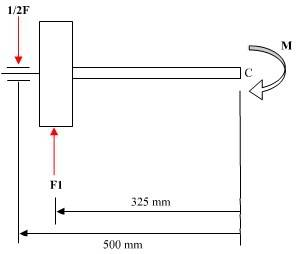# Bending moment

Calculate the bending moment in the centre of the axle??
What limit stress is valid for this loading case ?

may i know how to form the moment eqn??
F=300N

picture - http://files.filefront.com//;5410274;;/

pls help
thanx

## Answers and Replies

Did you show your work? Homework helpers will not assist with any questions until you've shown your own effort on the problem. Remember, we help with homework, we don't do your homework. We already passed those classes; it's your turn to do so.

What do you think? Try to form the equation on your own and we will inspect it.

i have try it many times but i don't know.
if in mechanics,this have to use the heaviside function,but this is machine design.therefore ,i don't know what to use.

i assume it is F(1) <x-175>^0 + F(1)<x-825^0>...
but i think this is incorrect

Well, heaviside functions aren't needed here.

Step 1: Determine the value of $$F_{1}$$ using equilibrium condition along the vertical (y-axis).

Step 2: By considering half part of the rotating machine (cut machine into two at the centre), write down the bending moment equation of either side using $$\sum\vec{F}l=\vec{M_{b}}$$. It will be the same for the other half since it is symmetrical.

Last edited:
if i cut into the half,then i'll get F * 325mm = M(b) rite??am i correct??

if it is correct,in order to find limit stress = bending moment / W , where W= pi (d^3) / 64 right??
how can i find the diameter and which shaft should i consider??Your answer isn't correct. Try writing down the moment at C. Then we discuss the rest of the question.

Last edited:
then it should be M + (F1*325) - (1/2F * 500) =0 right??am i correct??

if it is correct,in order to find limit stress = bending moment / W , where W= pi (d^3) / 64 right??
how can i find the diameter and which shaft should i consider??

Bingo.

How did you obtain the W? The correct axial section modulus W is $$W=\frac{\pi*d^3}{32}$$, assuming that the cross section of the axle is not hollow.

Setting $$\sigma_{all}=\frac{\sigma_{lim}}{n_{s}}=\frac{M_{b}}{W_{by}}$$ and solve for the diameter d as you have found $$M_{b}$$ in the first part, $$\sigma_{lim}$$ can be found in a strength data table, depending on the material given and the safety factor should be, well, leave the diameter in terms of $$n_{s}$$ if it isn't stated.

Last edited:
ok......thanx...pls help me in the other question in the engineering side...thanx